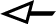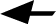In short, I study pattern formation in neural field models. There is a little introduction of these two terms, before describing my work (skip freely to the third paragraph).
Neural field models
In recent years significant progress has been made in understanding brain function by mathematical techniques - on the level of a single neuron dynamics, networks of neurons as well as at the level of neural tissue. Brain cortex could be thought of as a 2-dimensional sheet of tissue of densely interconnected neurons through which propagate spatially-structured impulses of neuronal activity. Thus, mean-field activity models could be applied to explain biological experiments showing activity synchronization throughout the brain, spread of waves or formation of stable patterns of activity. These are non-local models, where the activity at each neuronal population depends on a weighted function of the signal inputs from all other population sites. Neurons communicate by series of spikes which here are averaged into population firing rates. These statistical approximations allow us to describe large-scale neural tissue dynamics (i.e. on the order of millimetres)
by continuous mathematical equations often called neural fields, firing rate or mean-field models. They are integro-differential equations involving a differential operator for the synaptic processing, spatial convolution terms for the neuronal input and connectivity, and a nonlinear processing function converting the input firing rates to an output one. A variety of features could be added such as delays, adaptation, etc. Unfortunately, many of the studies of neural fields have concentrated on the biologically unrealistic local excitation-distal inhibition connectivity in order to obtain pattern solutions, an emphasis that we will try to balance.
Pattern formation
The models described above show very rich behaviour of solutions - travelling waves and fronts, bumps, breathers and globally periodic patterns. One part of my research has been to study periodic solutions using the theory of regular pattern formation. It was originally developed in physics to study (for example) convection, and dealt with PDEs. The typical scenario is that we start with a stable homogeneous solution and look for parameters at which the linear analysis tells us it becomes unstable to periodic spatial perturbations, the so called Turing instability.
This would lead to growth of inhomogeneous solutions i.e. patterns. However we have no information if the growth will saturate to a finite stable pattern and what will be its final form. The theory of pattern formation exploits the system symmetries to predict the geometry of possible patterns, and further, for parameters near the bifurcation point uses asymptotic expansions to derive the normal form of the bifurcation (amplitude equations). It governs the nonlinear selection between patterns that we will observe in the full system. Typically one would arrive at a system of Ginzburg-Landau equations.
A different approach is necessary to study localised patterns such as bumps or breathers. For these one can borrow on the techniques developed in nonlinear PDE systems for dispersive solitons, fronts and interfaces.
Research description
In view of the oscillatory patterns obtained by some authors in (one-population) scalar neural fields, we were interested in constructing the normal form for a Turing-Hopf instability in this context. We wanted to investigate what model features are needed to obtain dynamic patterns as opposed to static ones, and to achieve patterns in models with realistic local inhibition - distal excitation (inverted Mexican hat) type of connectivity.
Further we were curious about the selection between travelling and standing waves. We have developed the weakly nonlinear analysis for a general system that encompasses the class of neural fields with time-dependent connectivities (for example incorporating delays). The relevant amplitude equations were shown to be the mean-field Ginzburg-Landau equations. The generality of our initial work allowed us to apply the results to a wide variety of models suggested in the literature, including extensions with adaptation and two-population models. The theoretical predictions have become the basis for a number of numerical codes that allow us to quickly investigate the parameter space of a new model and, using both the linear and weakly non-linear instability analysis, plot the boundaries of parametric regions in which the homogeneous solution, a static pattern, travelling wave, standing wave or homogeneous (bulk) oscillation is preferred. All models are carefully checked by simulation of the full equations.
We extended our work to multi-population planar neural fields. We were able to derive an exact PDE equivalent form for the model with axonal delays which enabled us to simulate the planar equation and verify the Turing instability analysis. Weakly nonlinear analysis was not pursued due to the large variety of possible patterns in the plane one has to pick. However we looked at a two-population model incorporating patchy connections defined by a regular lattice.
Current and future work
Recently we shifted focus to a neural field with a Heaviside processing function possesing a dynamic threshold. This is a novel model taking into account that neuronal thresholds accommodate to persistent high input making the neurons less responsive to it. This mechanism is a crucial tool of computation in the nervous system. Simulations of the model exhibited a variety of very interesting localised dynamics reminiscent of dispersive solitons in the physical literature - stationary and travelling bumps and breathers, self-replicating dynamics and particle-like scattering between bumps, fingering instability and labirynth formation. We have begun to tackle analytically these intriguing phenomena by adapting weakly nonlinear pulse interaction, Amari technique and interface dynamics.